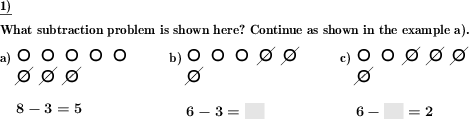Custom math worksheets at your fingertips# Details for problem "Subtraction term graphical representation"

Quickname: 8490

Elementary School, Primary School.

## Summary

A series of marbles represents a subtraction term - empty spaces to be filled in term.

## Example## Description

A subtraction term is illustrated in the form of two row of marbles with five each:

The minuend is represented by a set of marbles. As the cardinality of the subtrahend is equivalent to the number of marbles that are taken away from the original set of marbles, these marbles are marked as striked out. The remaining marbles stand for the difference that is left when the subtrahend is taken away from the minuend.

Below the graphical representation of the subtraction term, the term itself is shown with blank spaces for the

• minuend,
• subtrahend or
• difference,

depending on the setting of the problem - there is an option to configure the spaces that will have to be filled in.

The number of problems presented can be configured. There is a practice mode for a single number, if chosen, this number will always be the minuend. Otherwise, all numbers will be chosen at random.

The first problem may be selected to be a sample problem, which will be presented with the answer.

Download free printable worksheets for this math problem here. The worksheet contains the problems only, the solution sheet includes the answers. Just click on the respective link.

•Worksheet 1Solution sheet with answers
•Worksheet 2Solution sheet with answers
•Worksheet 3Solution sheet with answers

If you can not see the solution sheets for download, they may be filtered out by an ad blocker that you may have installed. If this is the case, please allow ads for this page and reload the page. The solution sheets will then reappear.

• Do these sample worksheets do not really fit?
• Do you need more math worksheets, with a different level of difficulty?
• Would you like to combine different problems on a worksheet and adjust them to your needs?
• As a teacher, you can put together your own worksheets using the automatically generated math problems provided.
With a free initial credit, you can start creating your own math worksheets in a few minutes.

You can try it for free! Register here, to create custom worksheets now!

## Customization options for this problem

Parameter
Possible values
Number of problems
1, 2, 3, 4, 5, 6, 7, 8, 9
Number to practice
All, 3, 4, 5, 6, 7, 8, 9, 10
Fill in...
subtrahend, difference, minuend, subtrahend,difference, minuend,subtrahend, minuend,difference, a random operand, two random operands, all operands
Sample problem
Yes, No

## Similar problems

Remark
Description
First steps to subtraction skills: Add dice pips so that sum of multiple dice is correct.
As a frames and arrows style calculation chain, successive application the same subtractive operation
Simple frames and arrows chain task with one elementary arithmetic operation, results are to be filled in blank spaces.